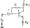# Electronic Circuits June-July 2007

Note : Answer any FIVE full questions.

1 a. Define diffusion capacitance. Derive an expression for the same.

b. Draw the piece wise linear V-I characteristics of a P-N junction diode. Give the circuit model for the ON state and OFF state.

2 a. The input voltage Vj to the two-level clipper circuit as shown in fig. 1(c) varies linearly from 0 to 150 V. Sketch the output voltage Vo to the time scale. Assume

diodes as ideal.

b. With necessary circuit and waveforms, explain a bridge rectifier circuit with capacitor filter. Derive an expression for the ripple factor.

3 a. Design a full wave rectifier filter to meet the following requirements. DC output voltage = 15 V, load resistance = 1 K, rms ripple voltage on capacitor <1 % of dc output voltage. The AC supply voltage is 230 V at 50 Hz.

b. What is the need for bias compensation? Explain the compensation techniques used for Vbe and Icbo*

The circuit shown in fig.3(b) uses silicon transistor with p = 45, Vcc ~ 24 V, Rc – 10 K* R – 10 K, Rg = 0.27 K. If Vce ~ 5 V under quiescent conditions, find the value of 4R? and the stability factor S(Ico)- & -fM:Fig.3(b)

c. What do you mean by thermal runway of a transistor? Explain.

4 a. Obtain an expression in terms of ‘h’ parameters for a transistor as a two-port network. Using the above developed equations obtain the hybrid model of CE, CC and CB configurations.

b.  State and explain Millers theorem.

c.  A transistor is connected as a common emitter amplifier driving a load of 10 MX It is supplied by a source of 1 kiQ internal resistance. The ‘h’ parameters are hie – 1.1 kCl, hfe = 50, hre = 2.5 X 10-4, hoe = —-—. Find:

i) Current gain

ii) Voltage

iii) Input impedance

iv) Output impedance.

5 a. Derive an expression for: i) Input conductance (gb’e) ii) Output conductance (gcc) for a transistor at high frequency.

b.  Give the classification of multistage amplifier. Explain the various distortions in amplifiers.

c.  Discuss the general characteristics of a negative feedback amplifier.

6 a. Derive an expression for input and output resistance of a voltage shunt feedback amplifier.

b.  Explain the different types of power amplifiers.

c.  An ideal class B- pushpull amplifier with input and output transformers, has VCc = 20 V, N2 = 2Ni and Rl = 10 £1 The transistors have Iife – 20. Let the input be sinusoidal. For the maximum output signal Vm = Vcc. Determine: i) The output signal power ii) Collector power dissipation iii) Conversion efficiency,

7 a. Obtain an expression for the closed loop gain of a non-inverting amplifier.

b.  With necessary sketch and characteristic curves explain the operation of a Schmitt trigger.

c.  What do you mean by precision rectifiers? Explain full wave precision rectifier.

8 a. Explain the working of SAR ADC.

b. Explain the working R – 2R ladder DAC.

c. Explain the applications of astable multivibrator as:

i) Square wave generator ii) To achieve variable duty cycle control.